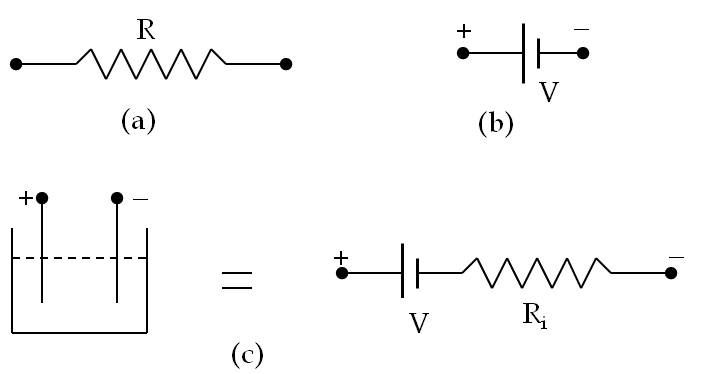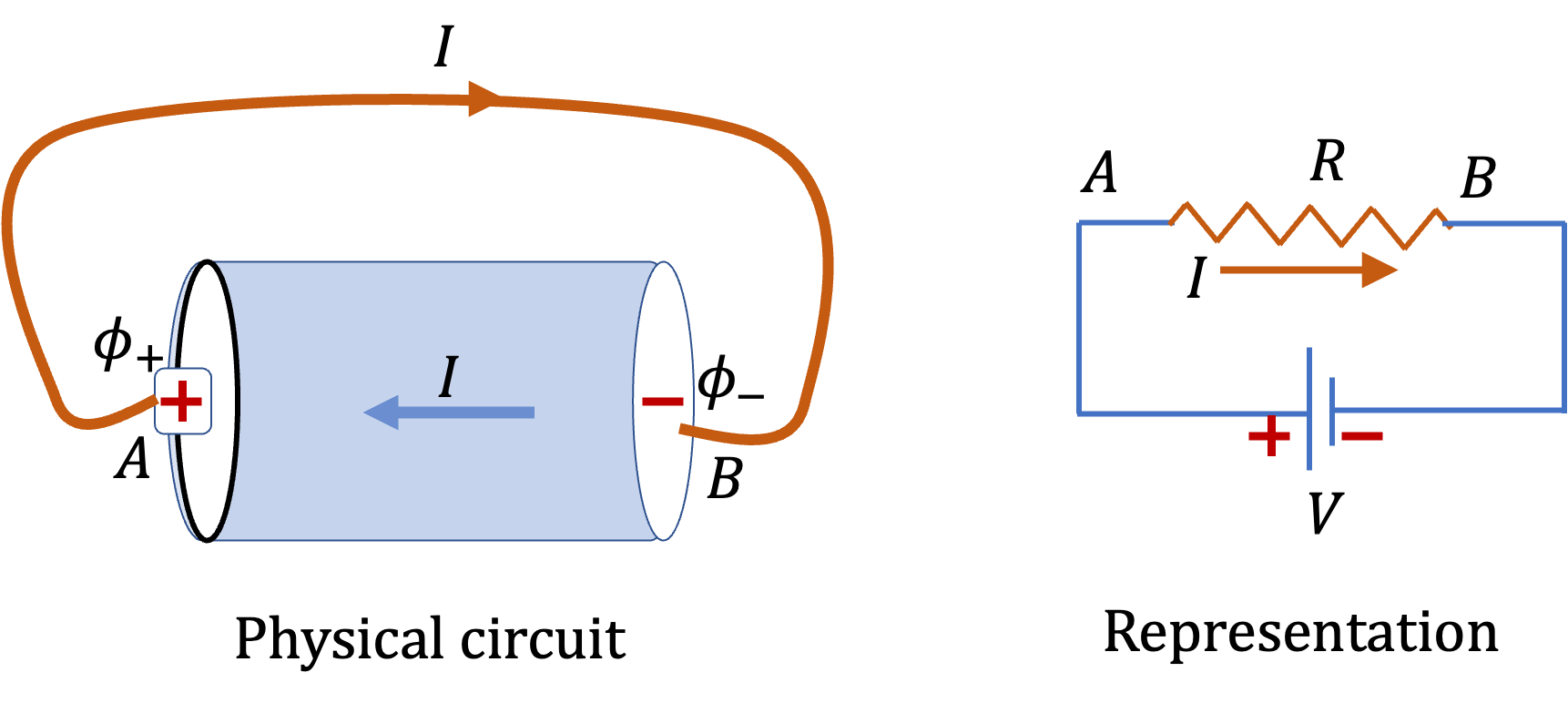## Section36.1Simple DC Circuits

A network of electrical devices connected together is called a circuit. A conductor used in a circuit that has a significant resistance is also called a resistor. When a circuit has only conductors and voltage sources, such as batteries, then the circuit is also called a resistive circuit. We will study circuits in which the potential difference between the terminals of the voltage sources does not change with time. These circuits are called direct-current (DC) circuits.

In a circuit diagram, a voltage source is usually represnted by two parallel unequal lines, with the higher potential end, i.e., the postitive terminal at longer line, and a resistor is represented by a saw tooth line as shown in Figure 36.1.1. We label circuit elements with values relevant to them, e.g., source is labeled with the voltage value and a resistor with the value of resistance.

Often batteries and other constant-voltage sources have significant resistance themselves, which adds to the total resistance of the circuit. The resitance of a source is also called the internal resistance. If the internal resistance is a significant factor in the circuit, we would include an internal resistance in the model as given in Figure 36.1.1(c), otherwise we will tend to ignore them.Figure 36.1.1. Representating circuit elements: (a ) resistor, (b) DC voltage source, and (c) an electrolytic DC voltage source with internal resistance. Voltage source is labeled with a voltage value that give the potential difference between the two terminals, $\Delta \phi = \phi_+ - \phi_- = V\text{.}$ The resistor is labeled with the value of resistance.

### Subsection36.1.1A Simple DC Circuit

When you connect a wire of resistance $R$ to the two terminals of a battery of constant voltege $V\text{,}$ as shown in Figure 36.1.2 you create potential difference across the resistor, whose value is $V\text{.}$

\begin{equation} \Delta \phi = V.\label{eq-potential-diff-across-wire-connected-to-battery}\tag{36.1.1} \end{equation}Figure 36.1.2. A wire of resistance $R$ is connected across the terminals of a battery of voltage $V\text{.}$ The potential drop across the resistor is $\Delta \phi = V\text{.}$ Ohm's law applied to the wire gives current through the wire as $I = V/R\text{.}$

When we apply Ohm's law to the resistor, we will get the following relation.

\begin{equation*} \Delta \phi = I R. \end{equation*}

Using Eq. (36.1.1) we get the following relation between the voltage of the battery and current through the wire.

\begin{equation} V = I R.\tag{36.1.2} \end{equation}

Now, we note that current is moving charges in the wire. Where do they go? Since charges are not accumulating any where, charges musl flow at the same rate inside the battery. Therefore, the current here is also current through the battery.

\begin{equation*} I_\text{inside battery} = I_\text{through wire} = \dfrac{V}{R}. \end{equation*}

The current through the single voltage source is also called overall current of the circuit.